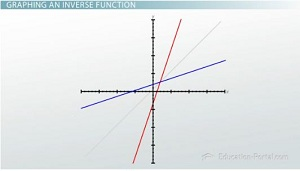# Understanding and Graphing the Inverse Function

An error occurred trying to load this video.

Try refreshing the page, or contact customer support.

Coming up next: Polynomial Functions: Properties and Factoring

### You're on a roll. Keep up the good work!

Replay
Your next lesson will play in 10 seconds
• 0:06 Inverse Functions
• 2:24 Five Steps to…
• 2:46 Examples
• 5:31 Graphing Inverse Functions
• 6:52 Lesson Summary
Save Save

Want to watch this again later?

Timeline
Autoplay
Autoplay
Speed Speed

#### Recommended Lessons and Courses for You

Lesson Transcript
Instructor: Robert Egan
If you use a function to map a to b, is there a way to go back from b to a again? Learn how to find and graph inverse functions so that you can turn a into b and back into a.

## Inverse Functions

If I tell you that I have a function that maps the number of feet in some distance to the number of inches in that distance, you might tell me that the function is y = f(x) where the input x is the number of feet and the output y is the number of inches. You might even tell me that

y = f(x) = 12x,

because there are 12 inches in every foot. But what if I told you that I wanted a function that does the exact opposite? What if I want a function to take the number of inches as input and return the number of feet as output? Could you tell me what this function is?

Inverse functions are exactly that. If we have a function

y = f(x),

then the inverse function is written as

y = f-1 (x),

and it does the exact opposite of the function. What happens if you put a function and its inverse into a composite function such as f-1 (f(x))? First, we evaluate the inner function, f(x), then we're going to evaluate the outer function f-1 (x).

Let's take a look at an example. Say we start with 4 feet. Well, our function is f(x) = 12x because there are 12 inches in every foot. If we plug in 4 feet to start, then

f(4) = 12 * 4 = 48 inches

Now if we take the inverse function, and the inverse function is going to be

f-1 (x) = x(1/12)

So, if we take 48 inches, then our inverse function,

f-1 (48) = 48 / 12 = 4 feet

Okay, so you might be able to find f(x) and f-1 (x) just based on your understanding of inches and feet, but how do you do it in general?

## Five Steps to Inversing a Function

1. Write your function out in terms of x and y: y = f(x).
2. Swap the x and y variables: x = f(y).
3. Solve for y as a function of x.
4. Set y = f-1 (x).
5. Check the composite function: f-1 (f(x)).

## ExamplesFollowing these steps, let's say we have a function f(x) = 3(x - 1) + 2.

We're going to write this out in terms of x and y:

y = 3(x - 1) + 2

Then we're going swap the x and y variables, so we're going to write this as:

x = 3(y - 1) + 2

This can be a confusing step if you're not careful, but at its heart, all you're doing is putting x everywhere you see y and putting y everywhere you see x. Then you're going to solve for y as a function of x. So I'm going to subtract 2 from both sides:

x - 2 = 3(y - 1),

divide both sides by 3:

(x - 2) / 3 = y - 1

and add 1 to both sides and I end up with:

y = 1 + (x - 2)/3

I'm going to call what's on the right-hand side my inverse function:

f-1 (x) = 1 + (x-2)/3

Finally, I'm going to check my answer, so I'm going to find

f-1 of (f(x))

To do this, I'm going to write

f(x) = 3(x-1) + 2

I'm going to plug that in as input for my inverse function, so

f-1 (x) = 1 + ((3(x-1) + 2) - 2)/3

I have my input here, so I'm just going to solve and simplify for

f-1 (x) = 1 + (3(x-1))/3: f-1 (x) = 1 + x - 1

To unlock this lesson you must be a Study.com Member.

### Register to view this lesson

Are you a student or a teacher?

#### See for yourself why 30 million people use Study.com

##### Become a Study.com member and start learning now.
Back
What teachers are saying about Study.com

### Earning College Credit

Did you know… We have over 200 college courses that prepare you to earn credit by exam that is accepted by over 1,500 colleges and universities. You can test out of the first two years of college and save thousands off your degree. Anyone can earn credit-by-exam regardless of age or education level.